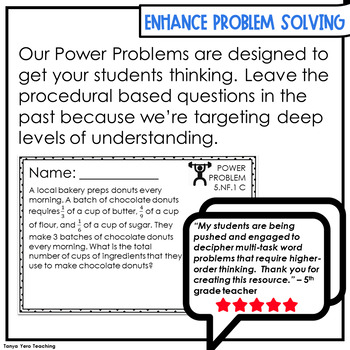# 5th Grade Word Problems Math | Math Spiral Review | Math Test Prep YEARLONG

Rated 4.87 out of 5, based on 547 reviews
547 Ratings;
5th
Subjects
Resource Type
Standards
Formats Included
• Zip
Pages
200 pages
\$12.50
List Price:
\$15.00
You Save:
\$2.50
Bundle
\$12.50
List Price:
\$15.00
You Save:
\$2.50
Bundle
Report this resource to TPTThis bundle contains one or more resources with Google apps (e.g. docs, slides, etc.).

#### What educators are saying

Tanya is my absolute go to for math resources. This fabulous resource really hones in on problem solving and is a great addition to any math program.
I used this with my higher level fifth graders. These problems really made them think and slow down when figuring the problem out. Really great product.

#### Bonus

POWER PROBLEM VIDEOS!
##### Also included in
1. The POWER Math Ultimate Bundle is everything you need for a successful year of math instruction! The resources found in this bundle were designed with the philosophy in mind that math should be POWERful. POWER stands for purposeful opportunities with engagement and rigor. You and your students deser
Price \$82.00Original Price \$111.70Save \$29.70

### Description

This set of 5th grade math word problems contains 5 questions for each math standard. The questions are designed to help build conceptual understanding.

These questions can be used for whole class lesson starters, 5th grade math small groups, rotations, homework, math spiral review and math test prep.

Math word problems help students take math understanding to the next level. And these Power Problems were designed to do just that. Not all math word problems are created equally, it is important that word problems are realistic, relatable and written to promote higher order thinking. Power Problems do just that!

Combining a math word problem attack strategy with a good set of math word problems is a proven research-based strategy for deepening students’ mathematical understanding.

You can confidently use these word problems knowing that the questioning is targeting conceptual understanding. Students will need to use their reading and comprehension skills while also applying everything they have learned in math class.

Mastering the art of solving math word problems takes a lot of practice. With five questions for each standard, this set of Power Problems includes plenty of opportunities for practice.

1. Display Math Applications in the Real World: Power Problems will show students how math concepts apply to real world situations and why math is so important.
2. Develop Critical Thinking Skills: Some basic reasoning and elimination skills can lead to success with multiple choice questioning, but these word problems will require students to read carefully, gather relevant information, solve the math problem and think about whether or not their answer makes sense in the context of the problem.
3. Application of Multiple Concepts: Power Problems will require students to pull apart problems, solve multiple pieces, put them back together into a logical solution.
4. Build Creativity: Procedural math questions don’t build a great deal of creativity. In fact, with enough procedural questioning, students will just memorize the answers. But with Power Problems, there will be opportunities for students to create their own equation to solve the problem leading to several different approaches.
5. Teacher Screening Aid: The best screeners of students are good teachers! And Power Problems are a great tool for teachers to visually see the mastery level of each of their students for each math standard or concept.

Standards & Topics Covered

Number and Operation in Base Ten

• 5.NBT.1 - Place value concepts
• 5.NBT.2 – Multiplying and dividing by powers of 10
• 5.NBT.3 - Number Form, Word Form, Expanded Form, and Comparing of decimals
• 5.NBT.4 – Rounding decimals
• 5.NBT.5 – Multiplying multi-digit numbers
• 5.NBT.6 - Dividing whole numbers
• 5.NBT.7 – Adding, subtracting, multiplying, and dividing decimals

Operations & Algebraic Thinking

• 5.OA.1 – Order of operations (including parenthesis, brackets, and braces)
• 5.OA.2 - Expressions
• 5.OA.3 – Patterns

Number and Operation - Fractions

• 5.NF.1 - Adding and subtracting fractions with unlike denominators
• 5.NF.2 – Fraction word problems
• 5.NF.3 – Connecting fractions with division
• 5.NF. 4 - Multiplying a fraction by a whole number or another fraction
• 5.NF.5 – Interpret multiplication as scaling
• 5.NF.6 – Fraction word problems including mixed numbers
• 5.NF.7 – Dividing fractions

Measurement and Data

• 5.MD.1 - Measurement and converting measurement with the customary and metric systems of length, weight, mass, liquid volume, and time
• 5.MD.2 – Line plots
• 5.MD.3 – Understanding volume
• 5.MD.4 – Measuring volume
• 5.MD.5 – Volume word problems

Geometry

• 5.G.1 – Understanding coordinate grids
• 5.G.2 – Interpreting coordinate grids
• 5.G.3 – Understanding the attributes of 2D shapes
Total Pages
200 pages
Included
Teaching Duration
1 Year
Report this resource to TPT
Reported resources will be reviewed by our team. Report this resource to let us know if this resource violates TPT’s content guidelines.

### Standards

to see state-specific standards (only available in the US).
Convert among different-sized standard measurement units within a given measurement system (e.g., convert 5 cm to 0.05 m), and use these conversions in solving multi-step, real world problems.
Make a line plot to display a data set of measurements in fractions of a unit (1/2, 1/4, 1/8). Use operations on fractions for this grade to solve problems involving information presented in line plots. For example, given different measurements of liquid in identical beakers, find the amount of liquid each beaker would contain if the total amount in all the beakers were redistributed equally.
Recognize volume as an attribute of solid figures and understand concepts of volume measurement.
A cube with side length 1 unit, called a “unit cube,” is said to have “one cubic unit” of volume, and can be used to measure volume.
A solid figure which can be packed without gaps or overlaps using 𝘯 unit cubes is said to have a volume of 𝘯 cubic units.